# Edge co-occurrences can account for rapid categorization of natural versus animal images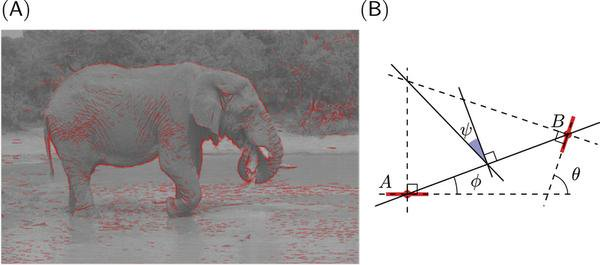### Abstract

Making a judgment about the semantic category of a visual scene, such as whether it contains an animal, is typically assumed to involve high-level associative brain areas. Previous explanations require progressively analyzing the scene hierarchically at increasing levels of abstraction, from edge extraction to mid-level object recognition and then object categorization. Here we show that the statistics of edge co-occurrences alone are sufficient to perform a rough yet robust (translation, scale, and rotation invariant) scene categorization. We first extracted the edges from images using a scale-space analysis coupled with a sparse coding algorithm. We then computed the association field” for different categories (natural, man-made, or containing an animal) by computing the statistics of edge co-occurrences. These differed strongly, with animal images having more curved configurations. We show that this geometry alone is sufficient for categorization, and that the pattern of errors made by humans is consistent with this procedure. Because these statistics could be measured as early as the primary visual cortex, the results challenge widely held assumptions about the flow of computations in the visual system. The results also suggest new algorithms for image classification and signal processing that exploit correlations between low-level structure and the underlying semantic category.

Type
Publication
Scientific Reports
• Press release
• communiqué de presse
• supplementary information
• supplementary material

# A study of how people can quickly spot animals by sight is helping uncover the workings of the human brain.

Scientists examined why volunteers who were shown hundreds of pictures - some with animals and some without - were able to detect animals in as little as one-tenth of a second. They found that one of the first parts of the brain to process visual information - the primary visual cortex - can control this fast response. More complex parts of the brain are not required at this stage, contrary to what was previously thought.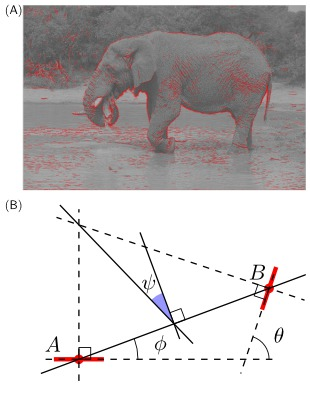Edge co-occurrences (A) An example image with the list of extracted edges overlaid. Each edge is represented by a red line segment which represents its position (center of segment), orientation, and scale (length of segment). We controlled the quality of the reconstruction from the edge information such that the residual energy was less than 5%. (B) The relationship between a reference edge A and another edge B can be quantified in terms of the difference between their orientations $\theta$, ratio of scale $\sigma$, distance $d$ between their centers, and difference of azimuth (angular location) $\phi$. Additionally, we define $\psi=\phi - \theta/2$, which is symmetric with respect to the choice of the reference edge; in particular, $\psi=0$ for co-circular edges. % (see text). As in~\citet{Geisler01}, edges outside a central circular mask are discarded in the computation of the statistics to avoid artifacts. (Image credit: Andrew Shiva, Creative Commons Attribution-Share Alike 3.0 Unported license). This is used to compute the chevron map in Figure~2.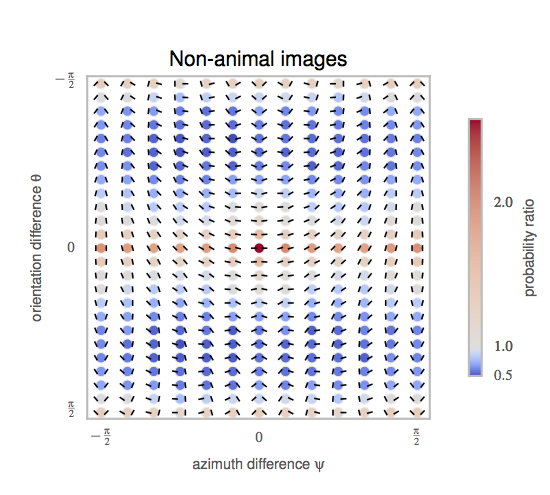The probability distribution function $p(\psi, \theta)$ represents the distribution of the different geometrical arrangements of edges’ angles, which we call a chevron map. We show here the histogram for non-animal natural images, illustrating the preference for co-linear edge configurations. For each chevron configuration, deeper and deeper red circles indicate configurations that are more and more likely with respect to a uniform prior, with an average maximum of about $3$ times more likely, and deeper and deeper blue circles indicate configurations less likely than a flat prior (with a minimum of about $0.8$ times as likely). Conveniently, this chevron map shows in one graph that non-animal natural images have on average a preference for co-linear and parallel edges, (the horizontal middle axis) and orthogonal angles (the top and bottom rows),along with a slight preference for co-circular configurations (for $\psi=0$ and $\psi=\pm \frac \pi 2$, just above and below the central row). We compare chevron maps in different image categories in Figure~3.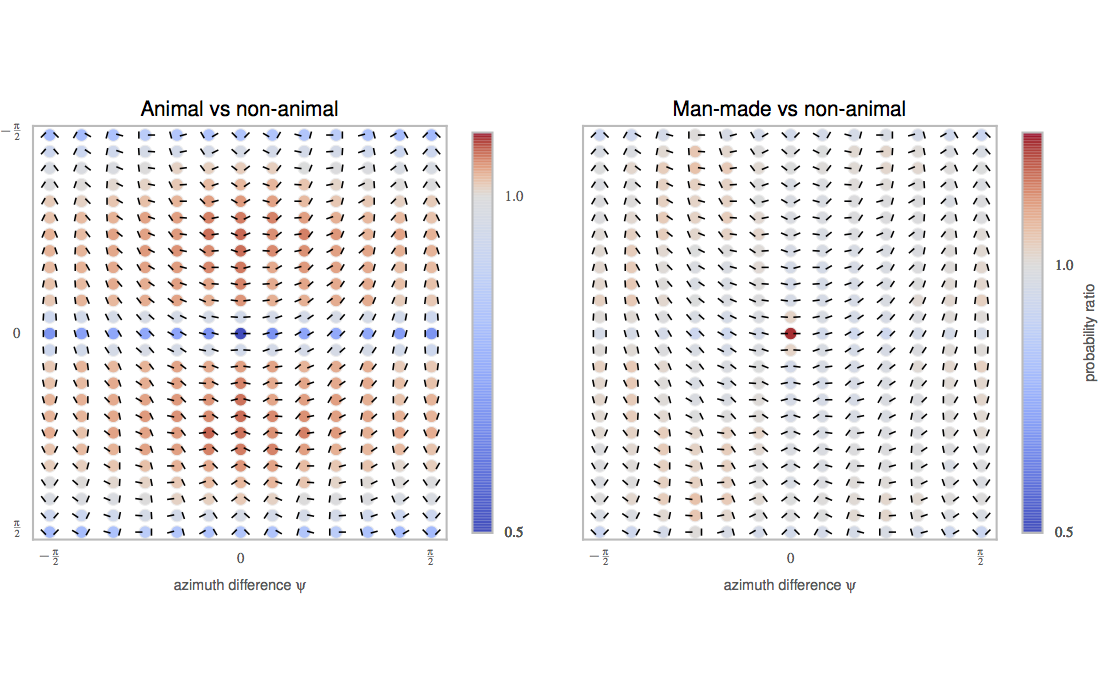As for Figure 2, we show the probability of edge configurations as chevron maps for two databases (man-made, animal). Here, we show the ratio of histogram counts relative to that of the non-animal natural image dataset. Deeper and deeper red circles indicate configurations that are more and more likely (and blue respectively less likely) with respect to the histogram computed for non-animal images. In the left plot, the animal images exhibit relatively more circular continuations and converging angles (red chevrons in the central vertical axis) relative to non-animal natural images, at the expense of co-linear, parallel, and orthogonal configurations (blue circles along the middle horizontal axis). The man-made images have strikingly more co-linear features (central circle), which reflects the prevalence of long, straight lines in the cage images in that dataset. We use this representation to categorize images from these different categories in Figure~4.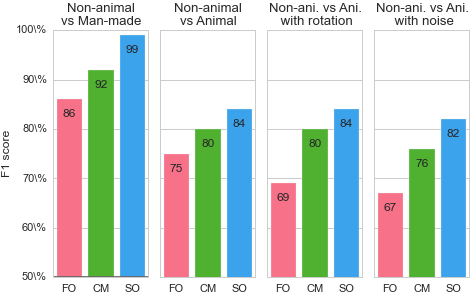Classification results. To quantify the difference in low-level feature statistics across categories (see Figure~3, we used a standard Support Vector Machine (SVM) classifier to measure how each representation affected the classifier’s reliability for identifying the image category. For each individual image, we constructed a vector of features as either (FO) the histogram of first-order statistics as the histogram of edges’ orientations, (CM) the chevron map subset of the second-order statistics, (i.e., the two-dimensional histogram of relative orientation and azimuth; see Figure 2 ), or (SO) the full, four-dimensional histogram of second-order statistics (i.e., all parameters of the edge co-occurrences). We gathered these vectors for each different class of images and report here the results of the SVM classifier using an F1 score (50\% represents chance level). While it was expected that differences would be clear between non-animal natural images versus laboratory (man-made) images, results are still quite high for classifying animal images versus non-animal natural images, and are in the range reported by~\citet{Serre07} (F1 score of 80\% for human observers and 82\% for their model), even using the CM features alone. We further extend this results to the psychophysical results of Serre et al. (2007) in Figure 5.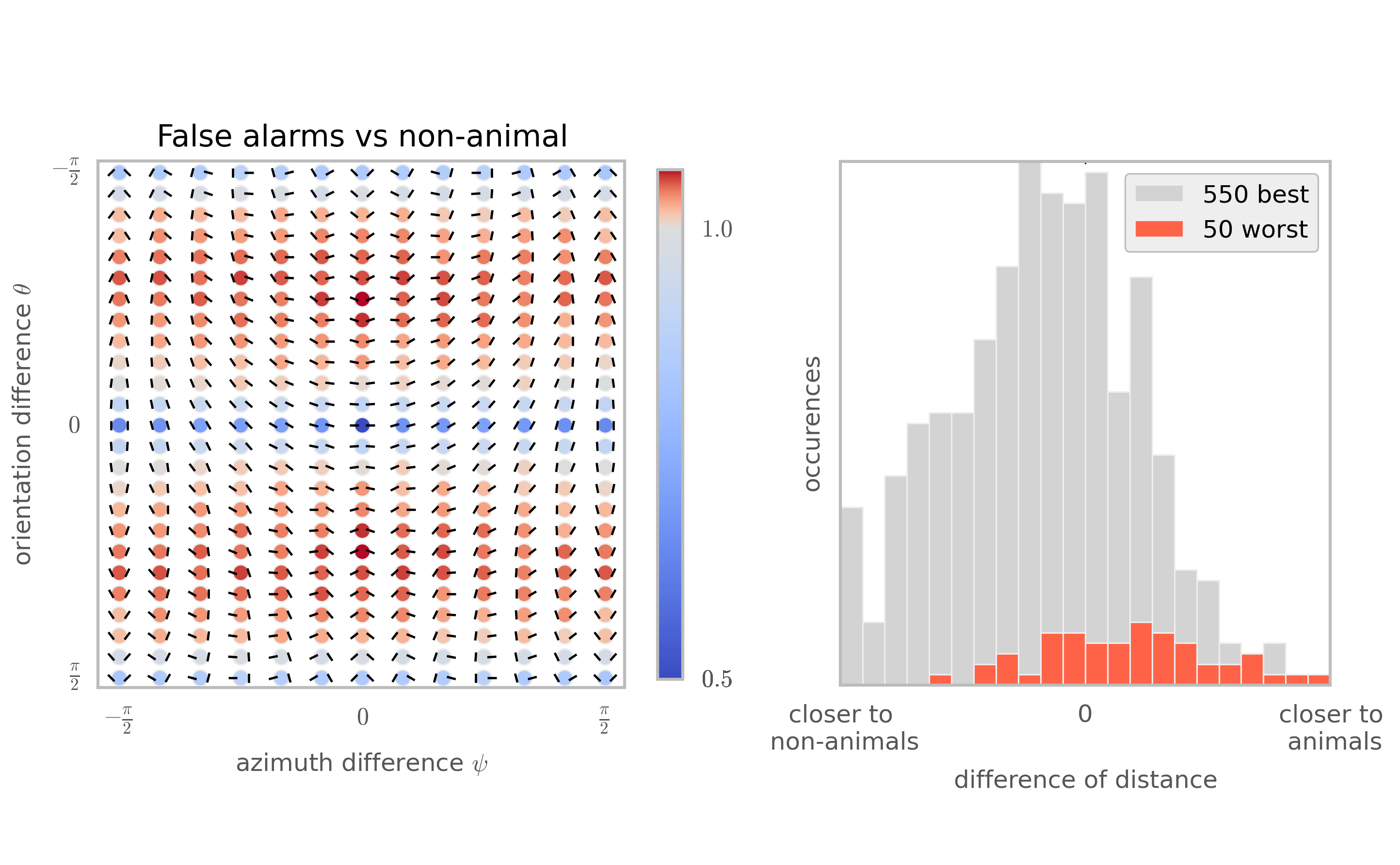To see whether the patterns of errors made by humans are consistent with our model, we studied the second-order statistics of the 50 non-animal images that human subjects in Serre et al. (2007) most commonly falsely reported as having an animal. We call this set of images the false-alarm image dataset. (Left) This chevron map plot shows the ratio between the second-order statistics of the false-alarm images and the full non-animal natural image dataset, computed as in Figure 3 (left). Just as for the images that actually do contain animals (Figure~3, left), the images falsely reported as having animals have more co-circular and converging (red chevrons) and fewer collinear and orthogonal configurations (blue chevrons). (Right) To quantify this similarity, we computed the Kullback-Leibler distance between the histogram of each of these images from the false-alarm image dataset, and the average histogram of each class. The difference between these two distances gives a quantitative measure of how close each image is to the average histograms for each class. Consistent with the idea that humans are using edge co-occurences to do rapid image categorization, the 50 non-animal images that were worst classified are biased toward the animal histogram ($d’ = 1.04$), while the 550 best classified non-animal images are closer to the non-animal histogram.##### Laurent U Perrinet
###### Researcher in Computational Neuroscience

My research interests include Machine Learning and computational neuroscience applied to Vision.